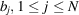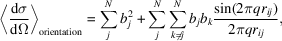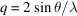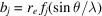International
Tables for
Crystallography
Volume H
Powder diffraction
Edited by C. J. Gilmore, J. A. Kaduk and H. Schenk

International Tables for Crystallography (2018). Vol. H, ch. 3.2, p. 261

## Section 3.2.4. The Debye scattering equation

P. W. Stephensa*

aDepartment of Physics and Astronomy, Stony Brook University, Stony Brook, NY 11794–3800, USA
Correspondence e-mail: peter.stephens@stonybrook.edu

### 3.2.4. The Debye scattering equation

| top | pdf |

The Debye scattering equation is an alternative method to compute the diffraction pattern of a collection of solid grains, which does not rely on a requirement of crystalline periodicity (Debye, 1915). Consequently, it is useful for cases where the grains of the sample are not fragments of idealized crystals, such as are frequently observed in nanoparticles. For a collection of identical grains, each containing N atoms having individual scattering amplitude, the orientation-average cross section per grain is given bywhereis the distance between atoms j and k, and the scattering vector. For neutrons, bj is the scattering length; in the case of multiple isotopes of a given atomic species, one may use the coherent scattering length to obtain the coherent elastic cross section. For X-rays,, the classical electron radius times the atomic scattering factor, and the cross section must be multiplied by the polarization factor as described in Section 3.2.2.2.1. This topic is treated in much greater detail in Chapters 3.6, 5.6and 5.7of this volume.

### References

Debye, P. (1915). Zerstreuung von Röntgenstrahlen. Ann. Phys. 351, 809–823.Google Scholar